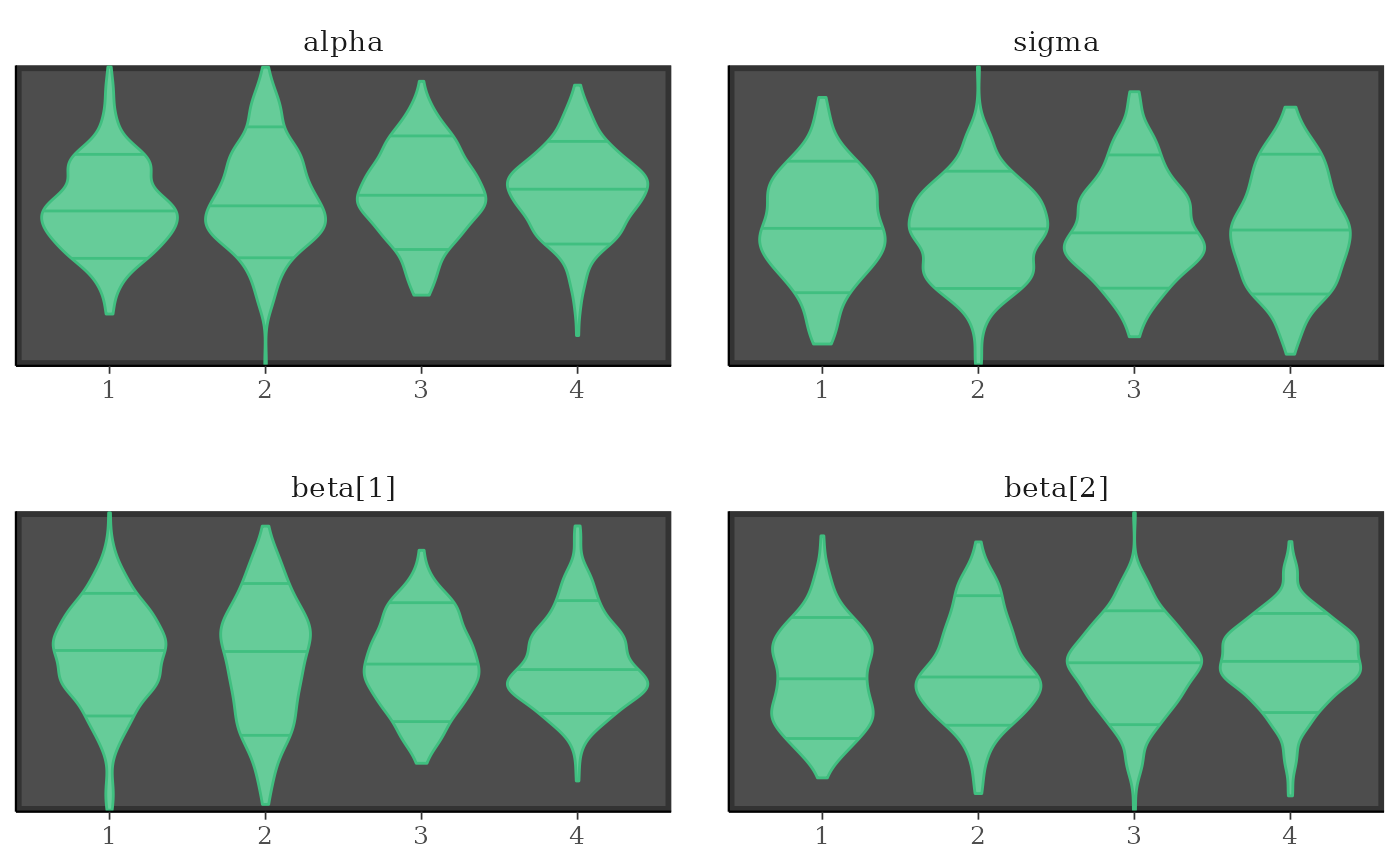Various types of histograms and kernel density plots of MCMC draws. See the Plot Descriptions section, below, for details.

mcmc_hist(
x,
pars = character(),
regex_pars = character(),
transformations = list(),
...,
facet_args = list(),
binwidth = NULL,
breaks = NULL,
freq = TRUE,
alpha = 1
)

mcmc_dens(
x,
pars = character(),
regex_pars = character(),
transformations = list(),
...,
facet_args = list(),
trim = FALSE,
bw = NULL,
kernel = NULL,
n_dens = NULL,
alpha = 1
)

mcmc_hist_by_chain(
x,
pars = character(),
regex_pars = character(),
transformations = list(),
...,
facet_args = list(),
binwidth = NULL,
freq = TRUE,
alpha = 1
)

mcmc_dens_overlay(
x,
pars = character(),
regex_pars = character(),
transformations = list(),
...,
facet_args = list(),
color_chains = TRUE,
trim = FALSE,
bw = NULL,
kernel = NULL,
n_dens = NULL
)

mcmc_dens_chains(
x,
pars = character(),
regex_pars = character(),
transformations = list(),
...,
color_chains = TRUE,
bw = NULL,
kernel = NULL,
n_dens = NULL
)

mcmc_dens_chains_data(
x,
pars = character(),
regex_pars = character(),
transformations = list(),
...,
bw = NULL,
kernel = NULL,
n_dens = NULL
)

mcmc_violin(
x,
pars = character(),
regex_pars = character(),
transformations = list(),
...,
facet_args = list(),
probs = c(0.1, 0.5, 0.9)
)

## Arguments

x

An object containing MCMC draws:

• A 3-D array, matrix, list of matrices, or data frame. The MCMC-overview page provides details on how to specify each these.

• A draws object from the posterior package (e.g., draws_array, draws_rvars, etc.).

• An object with an as.array() method that returns the same kind of 3-D array described on the MCMC-overview page.

pars

An optional character vector of parameter names. If neither pars nor regex_pars is specified then the default is to use all parameters. As of version 1.7.0, bayesplot also supports 'tidy' parameter selection by specifying pars = vars(...), where ... is specified the same way as in dplyr::select(...) and similar functions. Examples of using pars in this way can be found on the Tidy parameter selection page.

regex_pars

An optional regular expression to use for parameter selection. Can be specified instead of pars or in addition to pars. When using pars for tidy parameter selection, the regex_pars argument is ignored since select helpers perform a similar function.

transformations

Optionally, transformations to apply to parameters before plotting. If transformations is a function or a single string naming a function then that function will be used to transform all parameters. To apply transformations to particular parameters, the transformations argument can be a named list with length equal to the number of parameters to be transformed. Currently only univariate transformations of scalar parameters can be specified (multivariate transformations will be implemented in a future release). If transformations is a list, the name of each list element should be a parameter name and the content of each list element should be a function (or any item to match as a function via match.fun(), e.g. a string naming a function). If a function is specified by its name as a string (e.g. "log"), then it can be used to construct a new parameter label for the appropriate parameter (e.g. "log(sigma)"). If a function itself is specified (e.g. log or function(x) log(x)) then "t" is used in the new parameter label to indicate that the parameter is transformed (e.g. "t(sigma)").

Note: due to partial argument matching transformations can be abbreviated for convenience in interactive use (e.g., transform).

...

Currently ignored.

facet_args

A named list of arguments (other than facets) passed to ggplot2::facet_wrap() or ggplot2::facet_grid() to control faceting. Note: if scales is not included in facet_args then bayesplot may use scales="free" as the default (depending on the plot) instead of the ggplot2 default of scales="fixed".

binwidth

Passed to ggplot2::geom_histogram() to override the default binwidth.

breaks

Passed to ggplot2::geom_histogram() as an alternative to binwidth.

freq

For histograms, freq=TRUE (the default) puts count on the y-axis. Setting freq=FALSE puts density on the y-axis. (For many plots the y-axis text is off by default. To view the count or density labels on the y-axis see the yaxis_text() convenience function.)

alpha

Passed to the geom to control the transparency.

trim

A logical scalar passed to ggplot2::geom_density().

Optional arguments passed to stats::density() to override default kernel density estimation parameters. n_dens defaults to 1024.

color_chains

Option for whether to separately color chains.

probs

A numeric vector passed to ggplot2::geom_violin()'s draw_quantiles argument to specify at which quantiles to draw horizontal lines. Set to NULL to remove the lines.

## Value

A ggplot object that can be further customized using the ggplot2 package.

## Plot Descriptions

mcmc_hist()

Histograms of posterior draws with all chains merged.

mcmc_dens()

Kernel density plots of posterior draws with all chains merged.

mcmc_hist_by_chain()

Histograms of posterior draws with chains separated via faceting.

mcmc_dens_overlay()

Kernel density plots of posterior draws with chains separated but overlaid on a single plot.

mcmc_violin()

The density estimate of each chain is plotted as a violin with horizontal lines at notable quantiles.

mcmc_dens_chains()

Ridgeline kernel density plots of posterior draws with chains separated but overlaid on a single plot. In mcmc_dens_overlay() parameters appear in separate facets; in mcmc_dens_chains() they appear in the same panel and can overlap vertically.

Other MCMC: MCMC-combos, MCMC-diagnostics, MCMC-intervals, MCMC-nuts, MCMC-overview, MCMC-parcoord, MCMC-recover, MCMC-scatterplots, MCMC-traces

## Examples

set.seed(9262017)
# some parameter draws to use for demonstration
x <- example_mcmc_draws()
dim(x)
#>  250   4   4
dimnames(x)
#> $Iteration #> NULL #> #>$Chain
#>  "chain:1" "chain:2" "chain:3" "chain:4"
#>
#> \$Parameter
#>  "alpha"   "sigma"   "beta" "beta"
#>

##################
### Histograms ###
##################

# histograms of all parameters
color_scheme_set("brightblue")
mcmc_hist(x)
#> stat_bin() using bins = 30. Pick better value with binwidth.# histograms of some parameters
color_scheme_set("pink")
mcmc_hist(x, pars = c("alpha", "beta"))
#> stat_bin() using bins = 30. Pick better value with binwidth.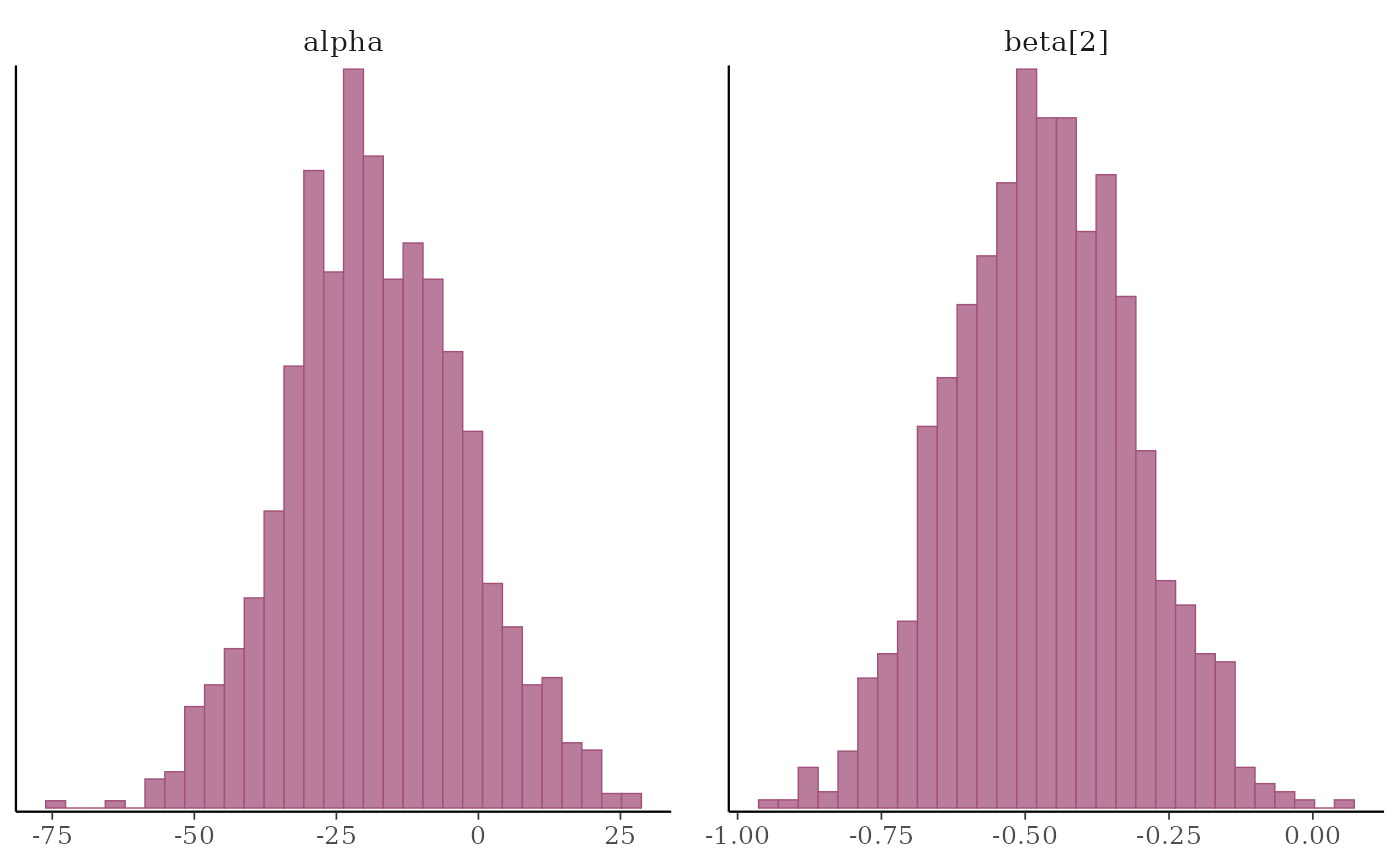# \donttest{
mcmc_hist(x, pars = "sigma", regex_pars = "beta")
#> stat_bin() using bins = 30. Pick better value with binwidth.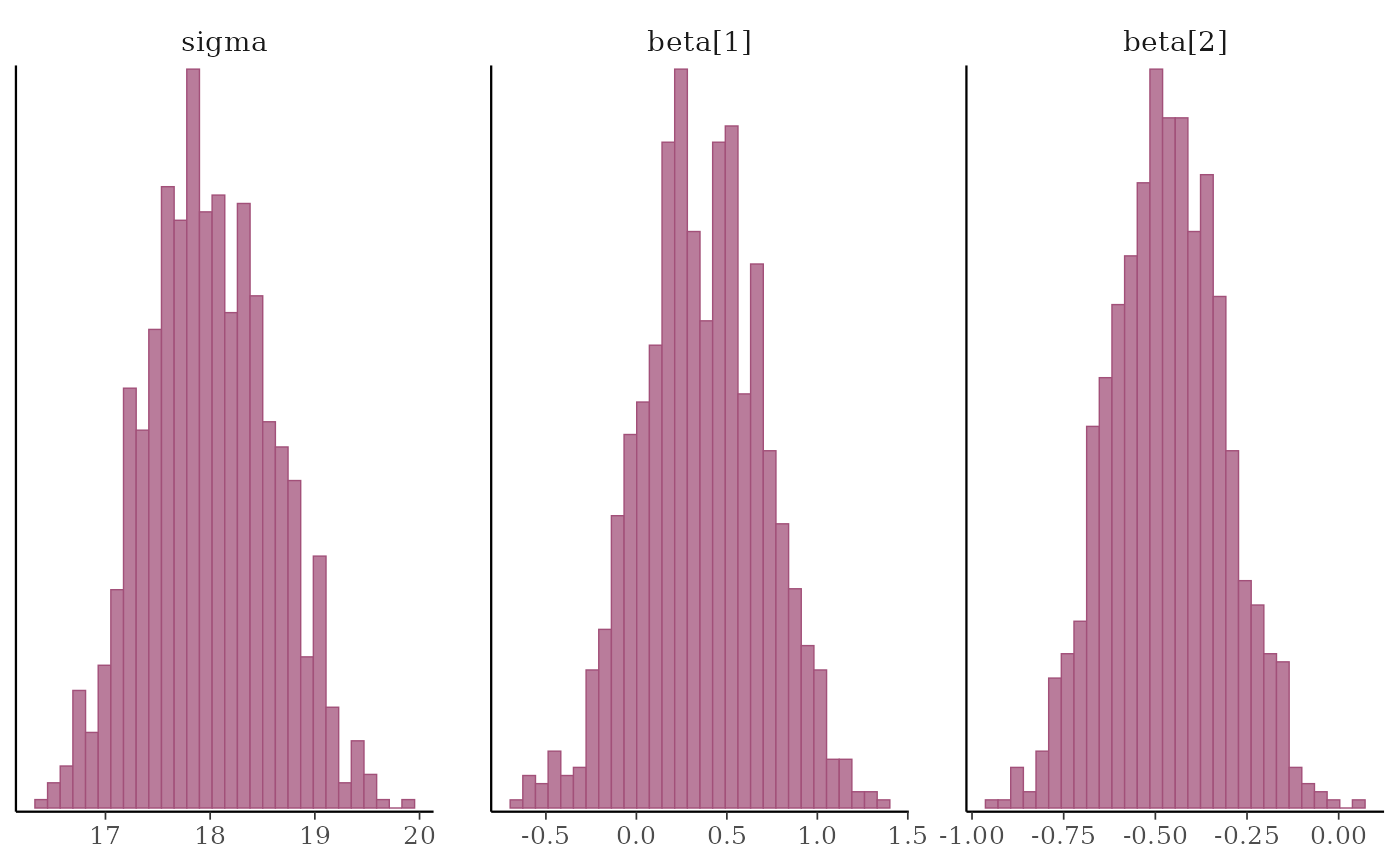# }
# example of using 'transformations' argument to plot log(sigma),
# and parsing facet labels (e.g. to get greek letters for parameters)
mcmc_hist(x, transformations = list(sigma = "log"),
facet_args = list(labeller = ggplot2::label_parsed)) +
facet_text(size = 15)
#> stat_bin() using bins = 30. Pick better value with binwidth.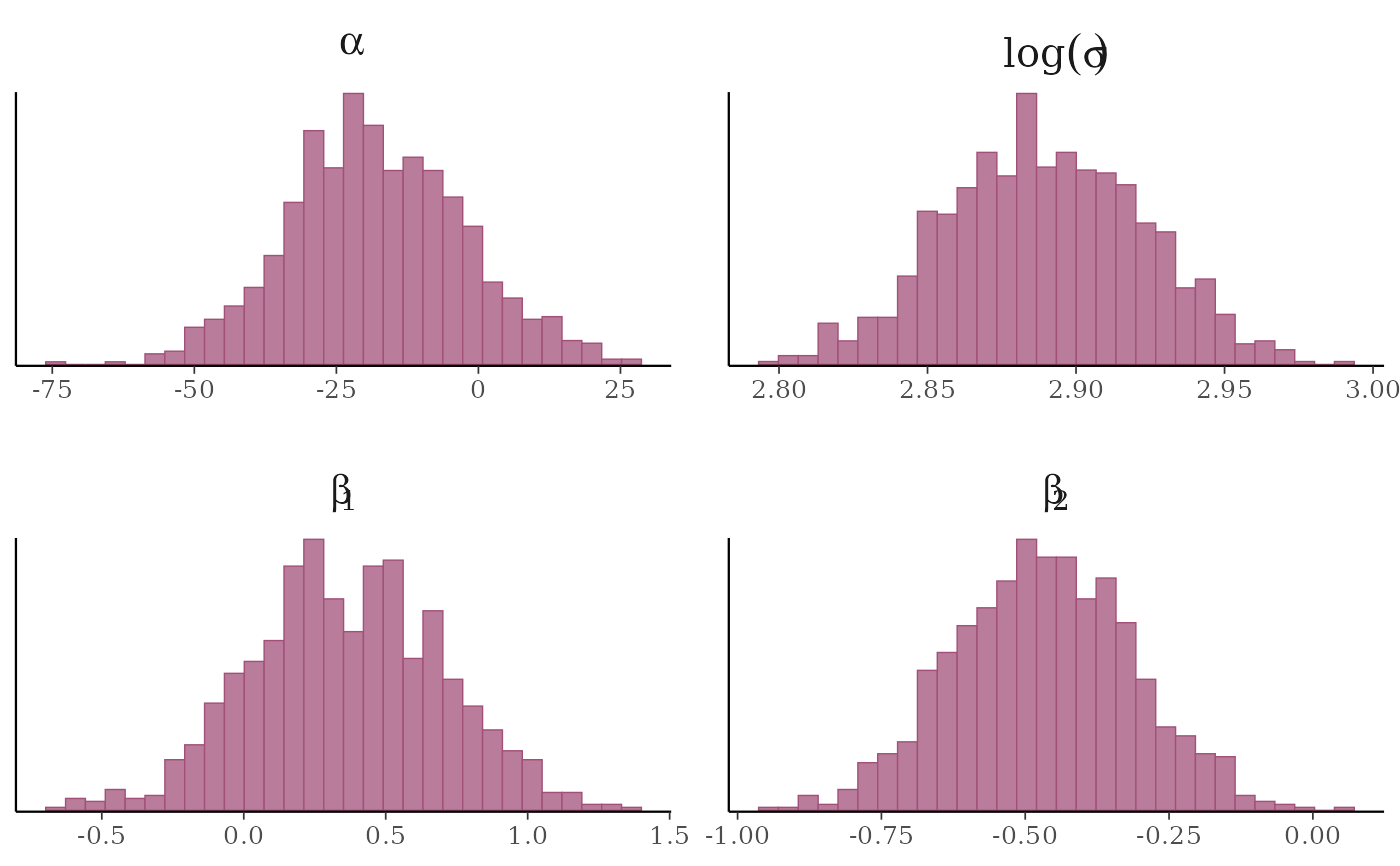# \donttest{
# instead of list(sigma = "log"), you could specify the transformation as
# list(sigma = log) or list(sigma = function(x) log(x)), but then the
# label for the transformed sigma is 't(sigma)' instead of 'log(sigma)'
mcmc_hist(x, transformations = list(sigma = log))
#> stat_bin() using bins = 30. Pick better value with binwidth.# separate histograms by chain
color_scheme_set("pink")
mcmc_hist_by_chain(x, regex_pars = "beta")
#> stat_bin() using bins = 30. Pick better value with binwidth.# }

#################
### Densities ###
#################

mcmc_dens(x, pars = c("sigma", "beta"),
facet_args = list(nrow = 2))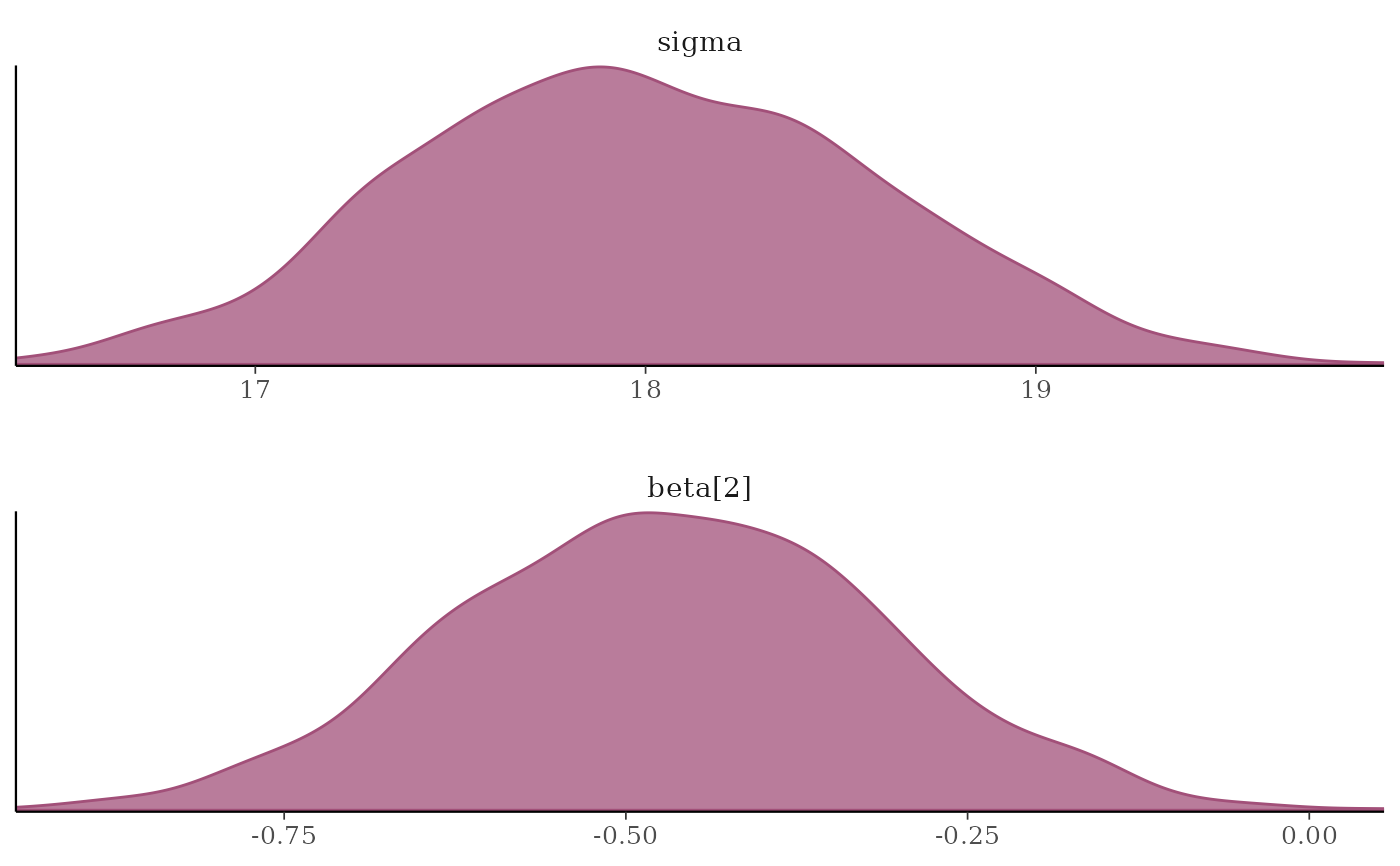# \donttest{
# separate and overlay chains
color_scheme_set("mix-teal-pink")
mcmc_dens_overlay(x, pars = c("sigma", "beta"),
facet_args = list(nrow = 2)) +
facet_text(size = 14)x2 <- example_mcmc_draws(params = 6)
mcmc_dens_chains(x2, pars = c("beta", "beta", "beta"))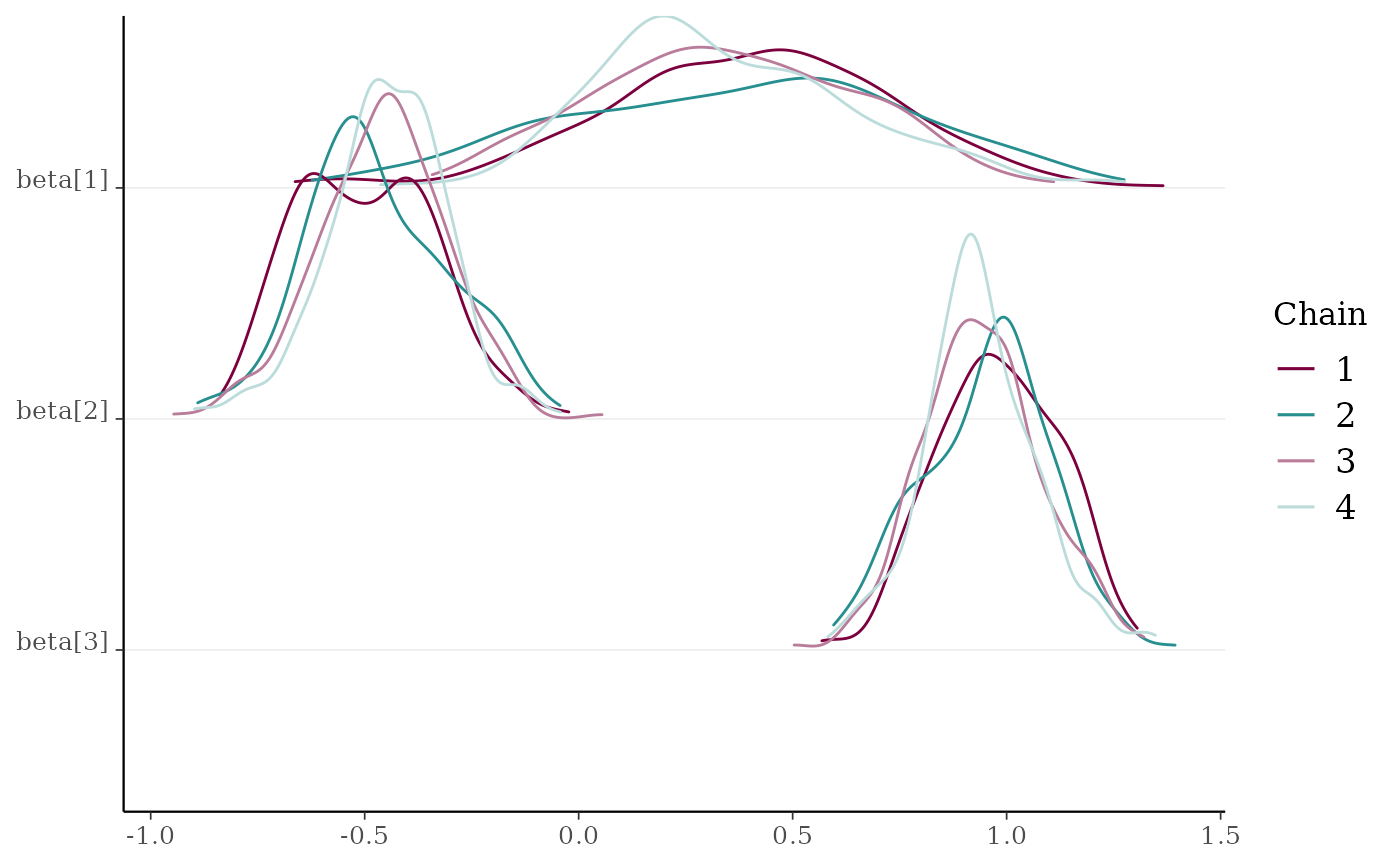# }
# separate chains as violin plots
color_scheme_set("green")
mcmc_violin(x) + panel_bg(color = "gray20", size = 2, fill = "gray30")